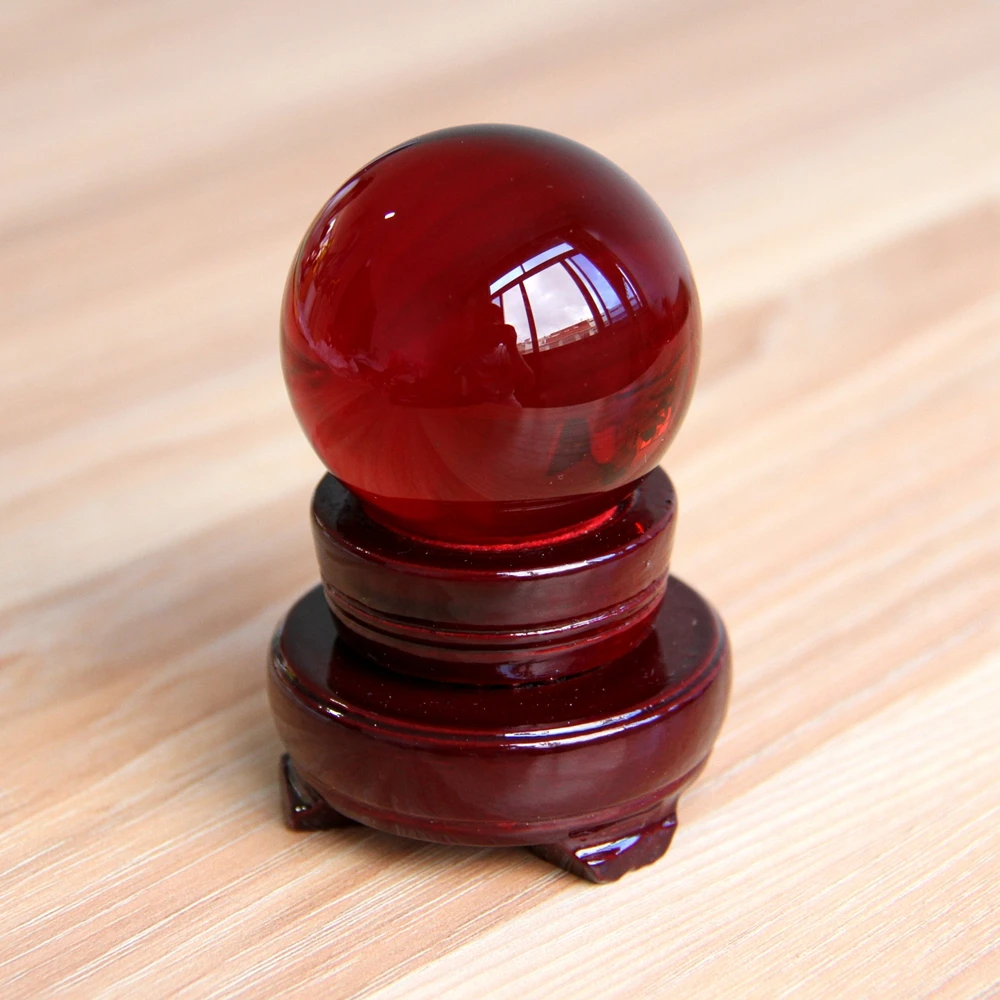# 60mm 1set red glass art crystal ball photography decorative wooden base glass balls for sale

\$26.37
Product Code:
1603003284695
Stock
Instock

### Overview

60mm 1set red glass art crystal ball photography   decorative wooden base glass balls for sale           Product Describtion  ^^^...
More Choices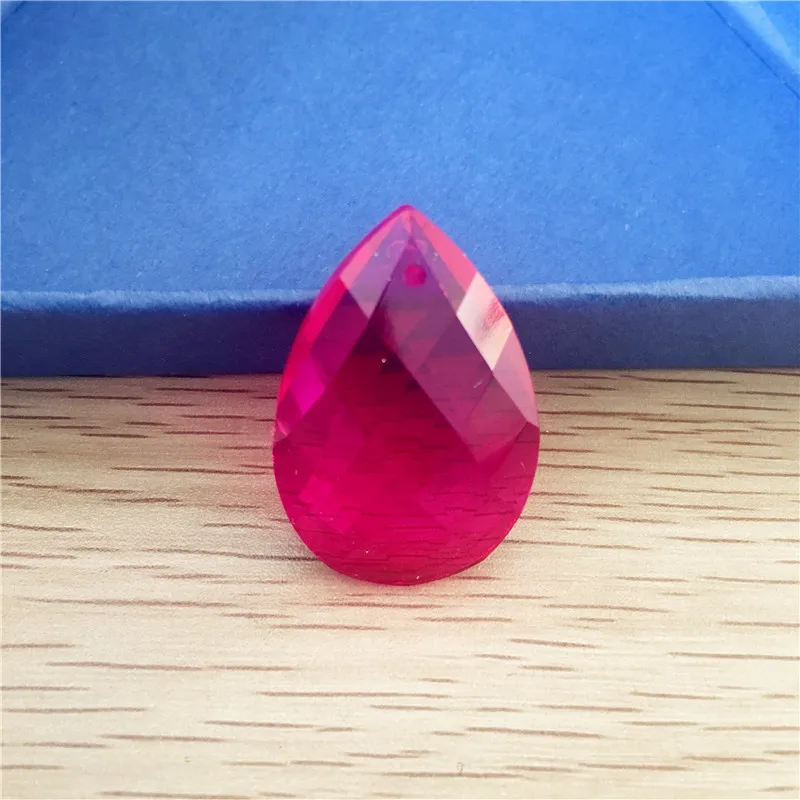US \$ 22.91 /Piece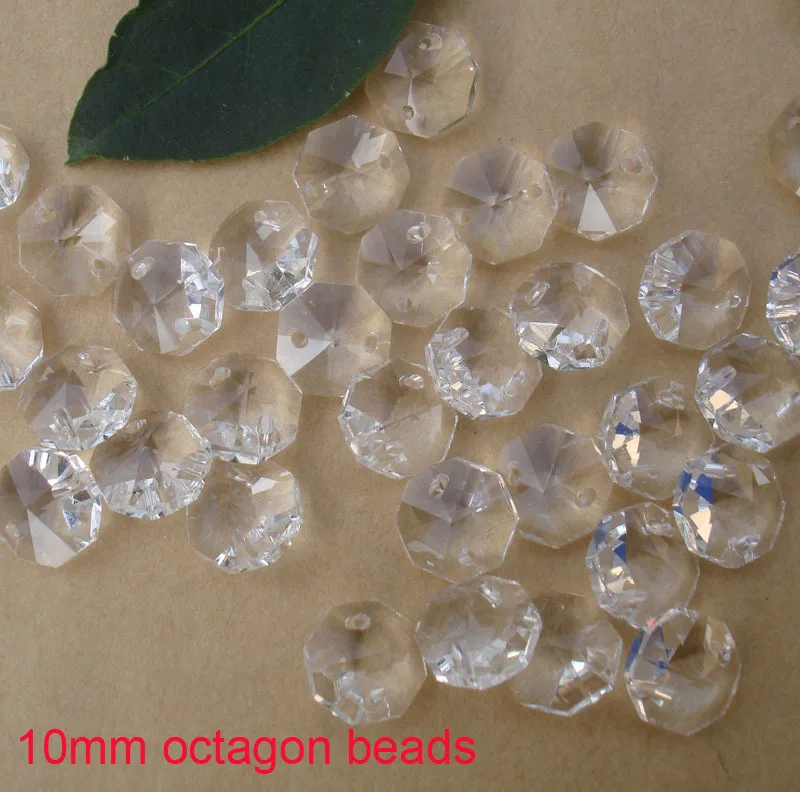US \$ 160.89 /Piece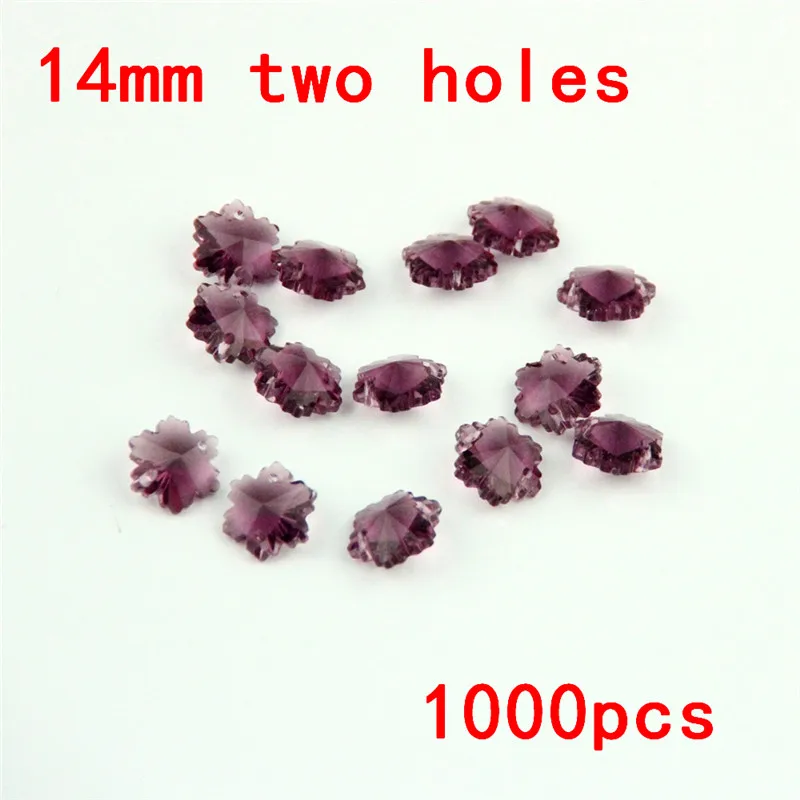US \$ 81.65 /PieceUS \$ 45.14 /Piece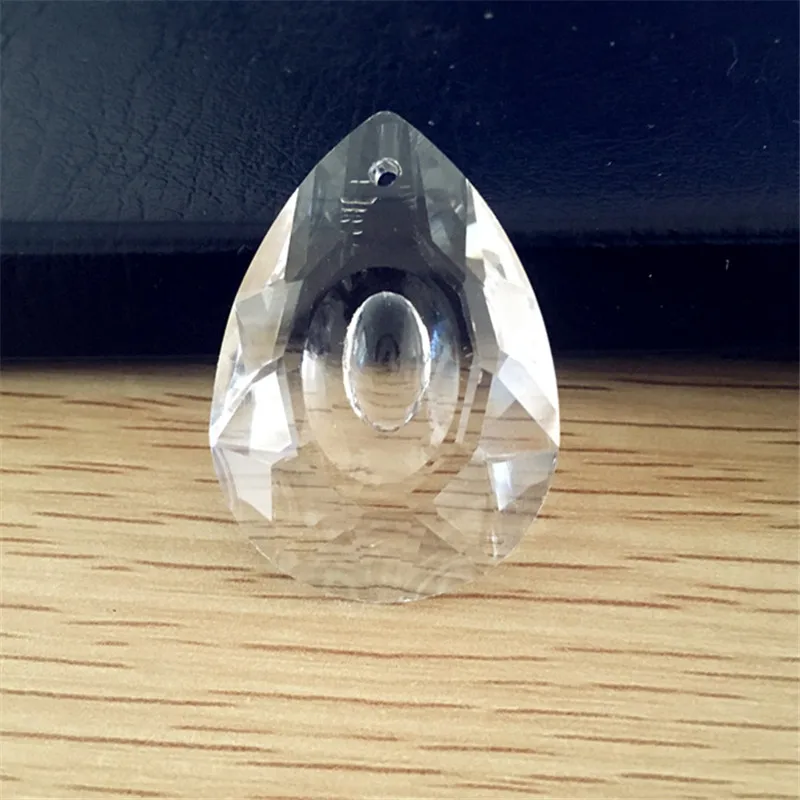US \$ 84.08 /Piece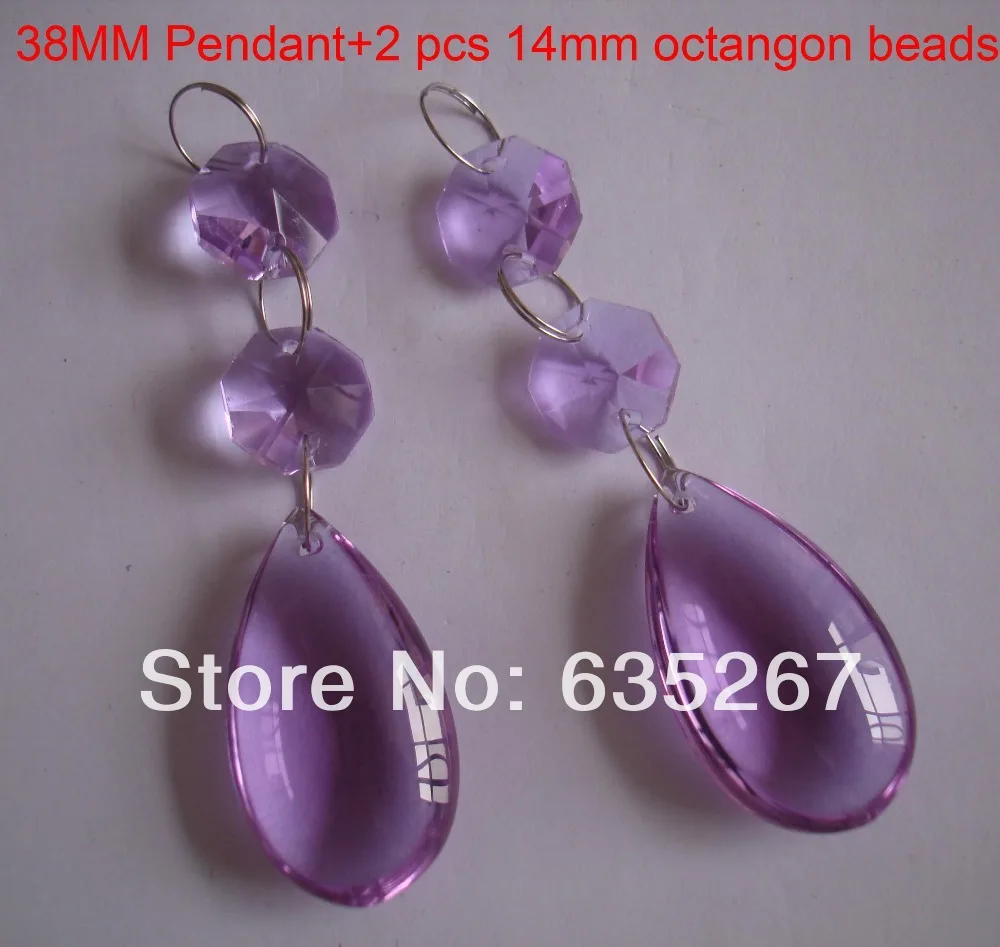US \$ 36.57 /Piece

60mm 1set red glass art crystal ball photography

decorative wooden base glass balls for sale

Product Describtion

^^^^^^^^^^^^^^^^^^^^^^^^^^^^^^^^^^^^^^^^^^^^^^^^^^^^^^^^^^^^^^^^^^^^^^^^^^^^^^^^^^^^^^^^^^^^^^^^^^^^^^^^^^^^^^^^^^^^^^^^

All the crystal in our store is made of high quality crystal glass ,not any arylic or palstic~

Size: 60mm  ( about 2.36 inch  )

Quantity : 1pieces ball with wooden  stand

Color : Red

Shape:round

Ball Net Weight :300 gram

Material: crystal glass

Other Size : 40mm, 50mm, 60mm, 70mm, 80mm, 100mm

Other Colors :Clear, Topaz, Green Blue ,Aquamrine

Red ,Lilac,Pink , Orange , Black

The taxes, VAT and other import duties/costs are NOT included in the Free shipping price which the customer pays the order for us .

More

^^^^^^^^^^^^^^^^^^^^^^^^^^^^^^^^^^^^^^^^^^^^^^^^^^^^^^^^^^^^^^^^^^^^^^^^^^^^^^^^^^^^^^^^^^^^^^^^^^^^^^^^^^^^^^^^^^^^^^^^

Detail Display

^^^^^^^^^^^^^^^^^^^^^^^^^^^^^^^^^^^^^^^^^^^^^^^^^^^^^^^^^^^^^^^^^^^^^^^^^^^^^^^^^^^^^^^^^^^^^^^^^^^^^^^^^^^^^^^^^^^^^^^^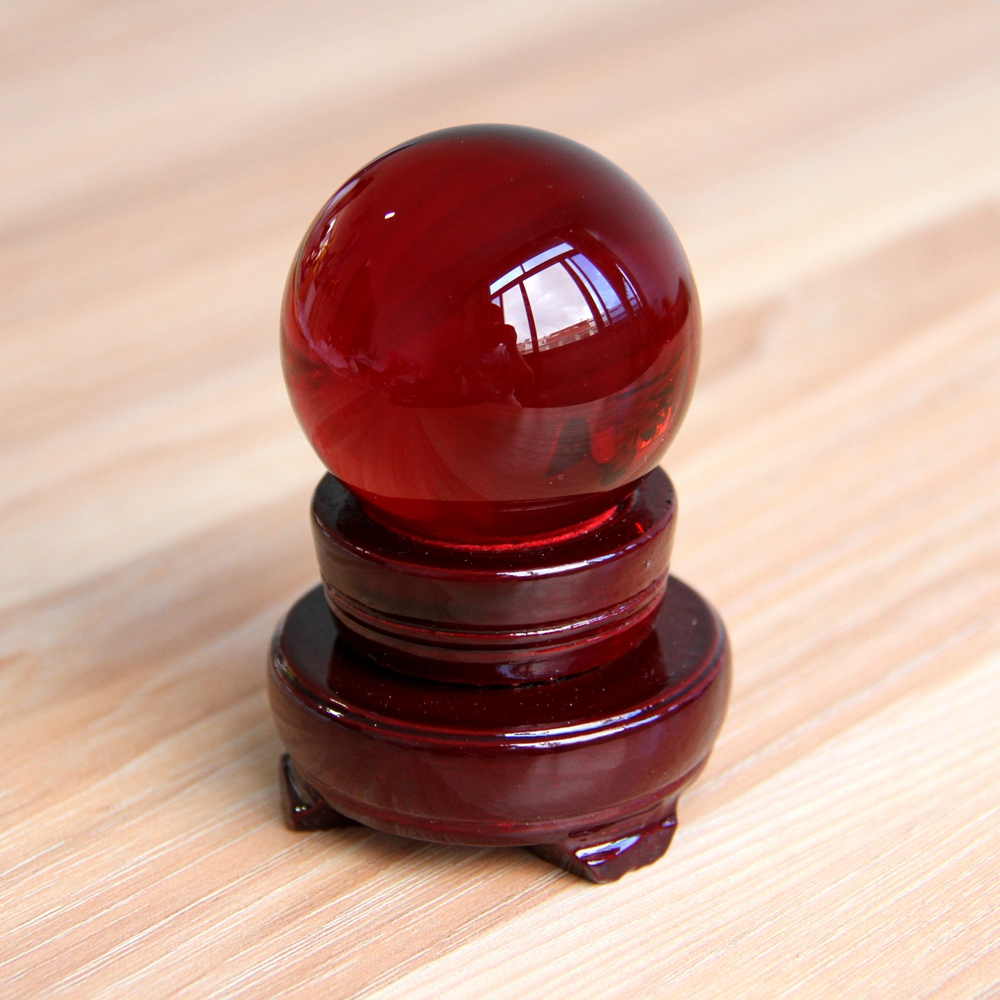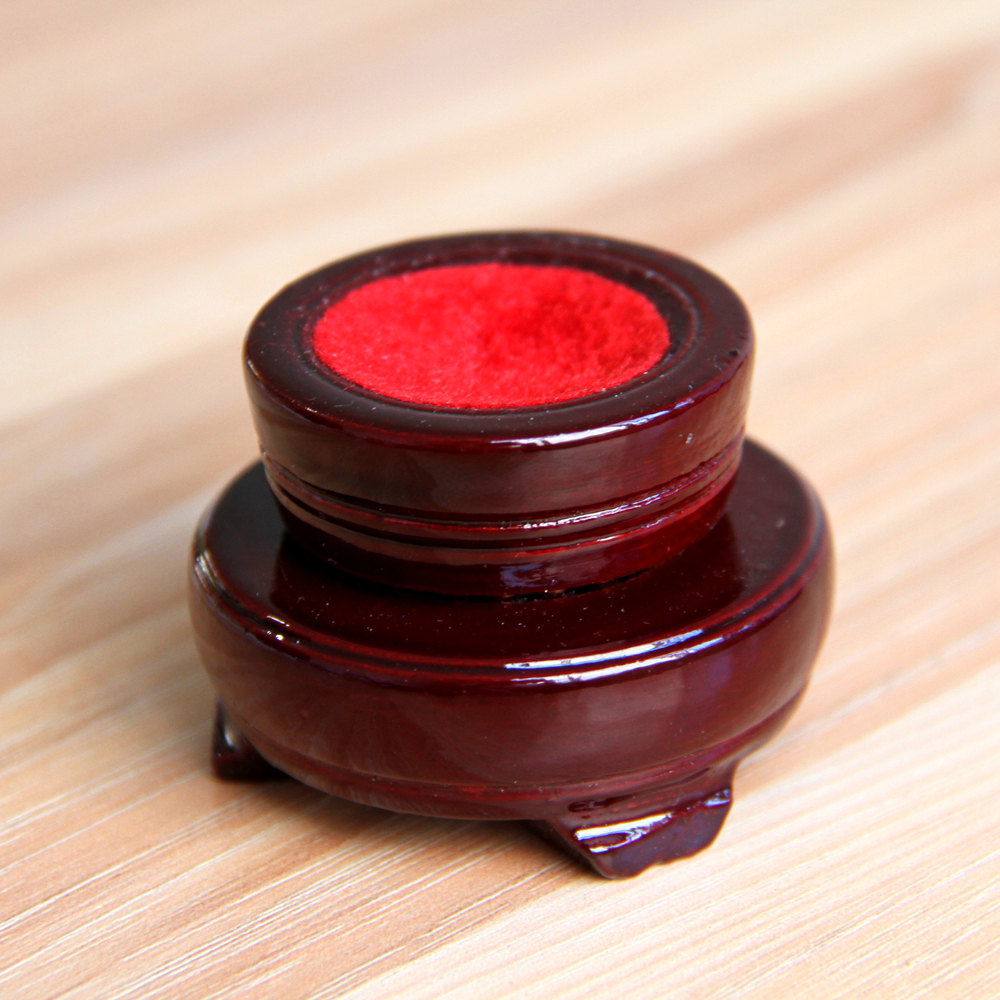Other Colors  ...........

^^^^^^^^^^^^^^^^^^^^^^^^^^^^^^^^^^^^^^^^^^^^^^^^^^^^^^^^^^^^^^^^^^^^^^^^^^^^^^^^^^^^^^^^^^^^^^^^^^^^^^^^^^^^^^^^^^^^^^^^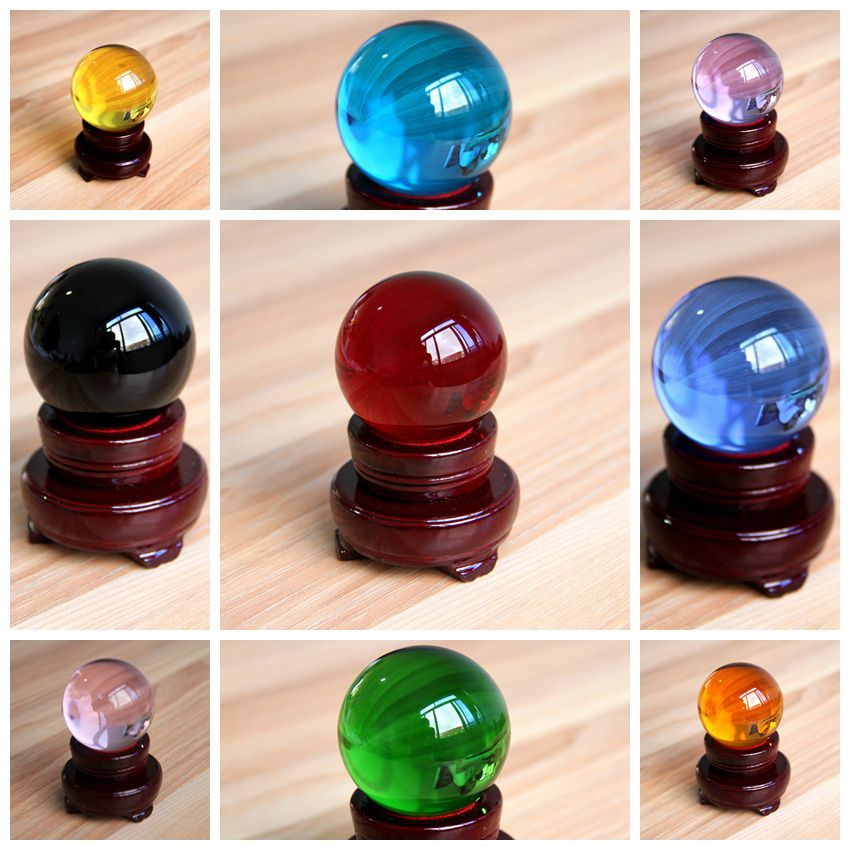Other Colors  ...........

^^^^^^^^^^^^^^^^^^^^^^^^^^^^^^^^^^^^^^^^^^^^^^^^^^^^^^^^^^^^^^^^^^^^^^^^^^^^^^^^^^^^^^^^^^^^^^^^^^^^^^^^^^^^^^^^^^^^^^^^

the below picture is 80mm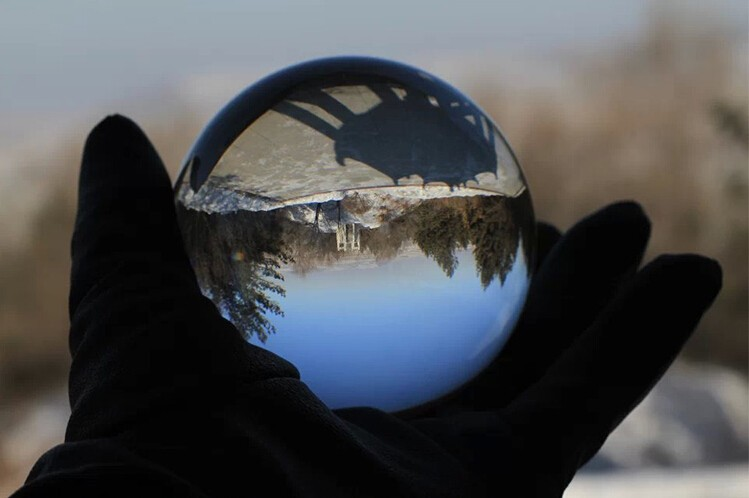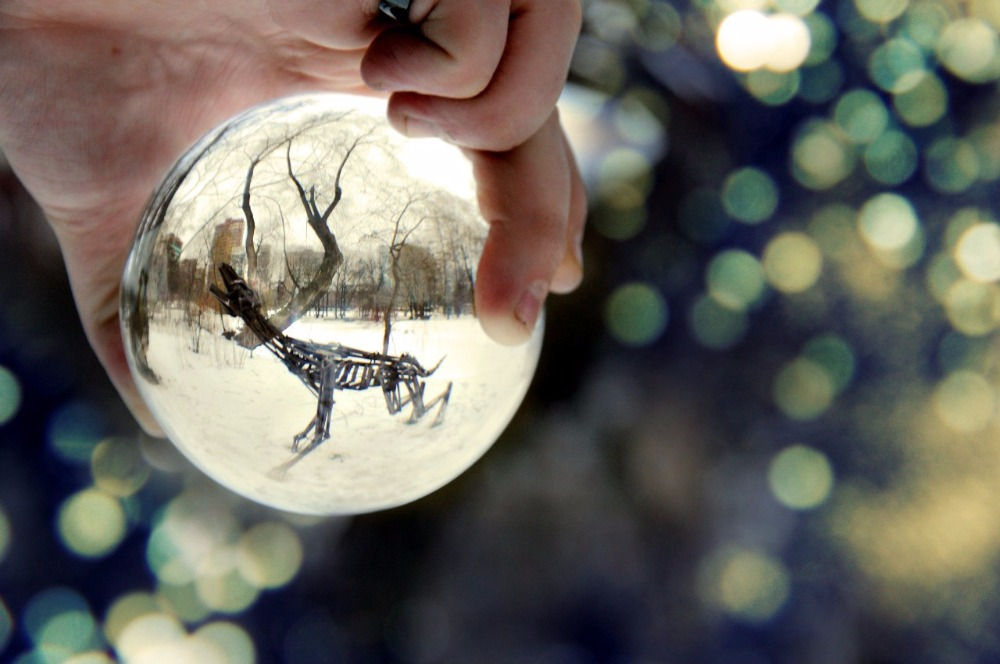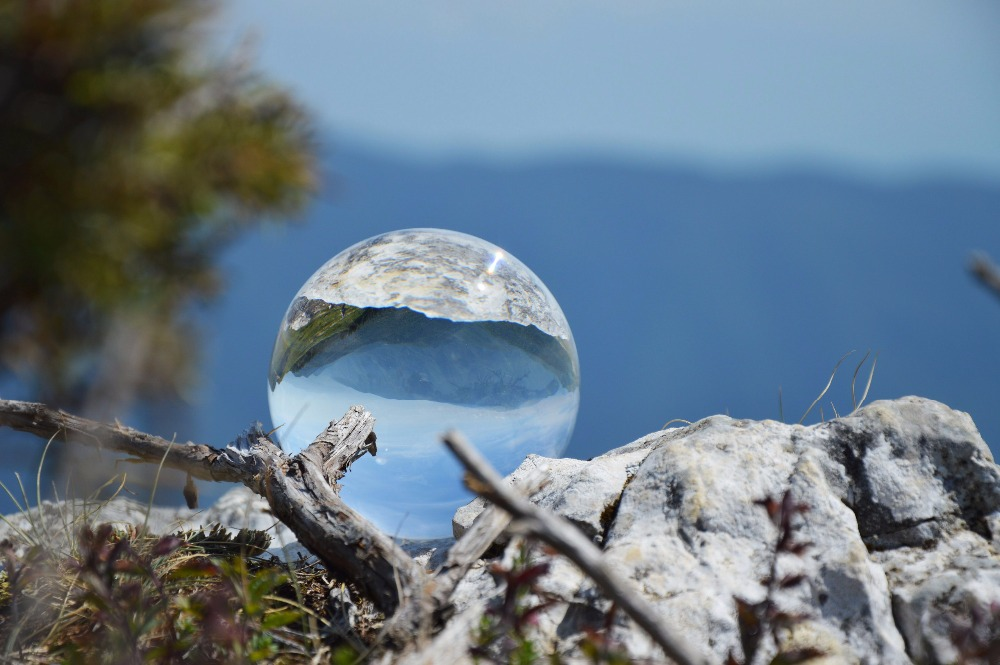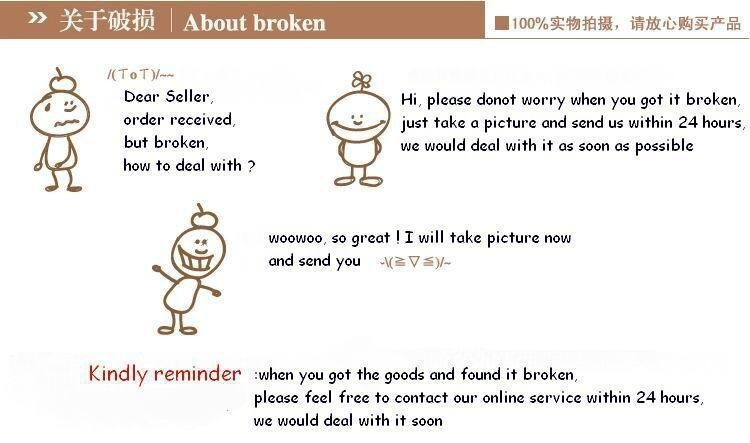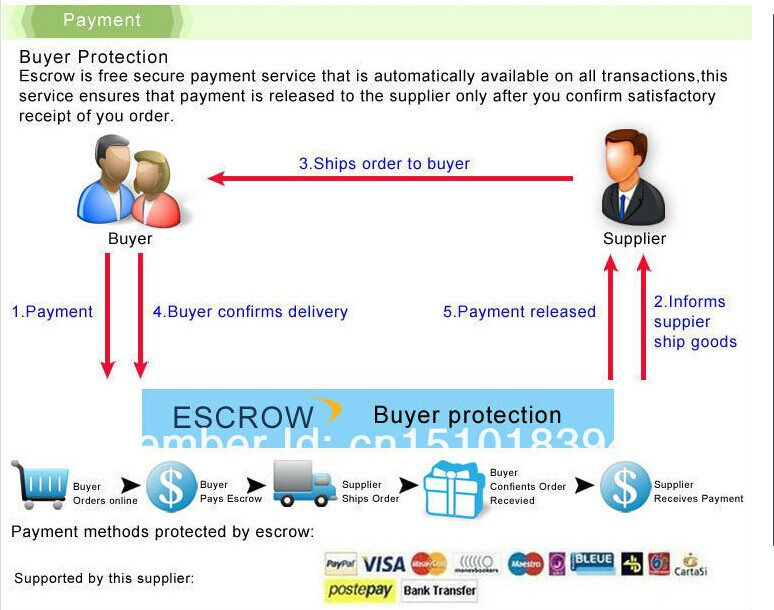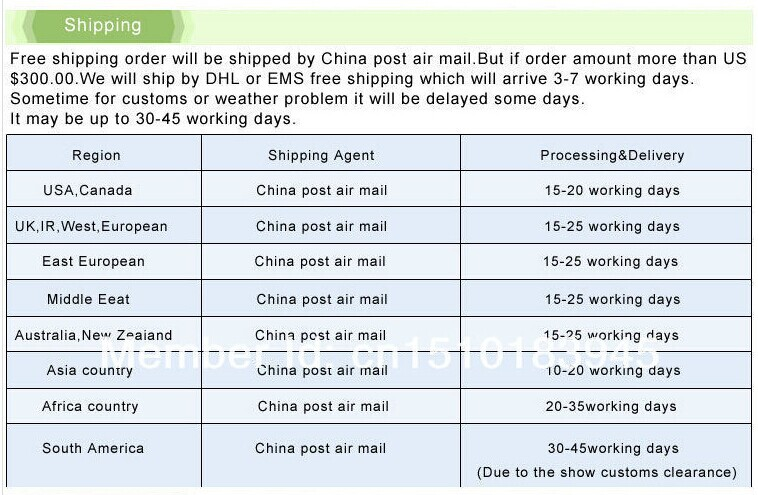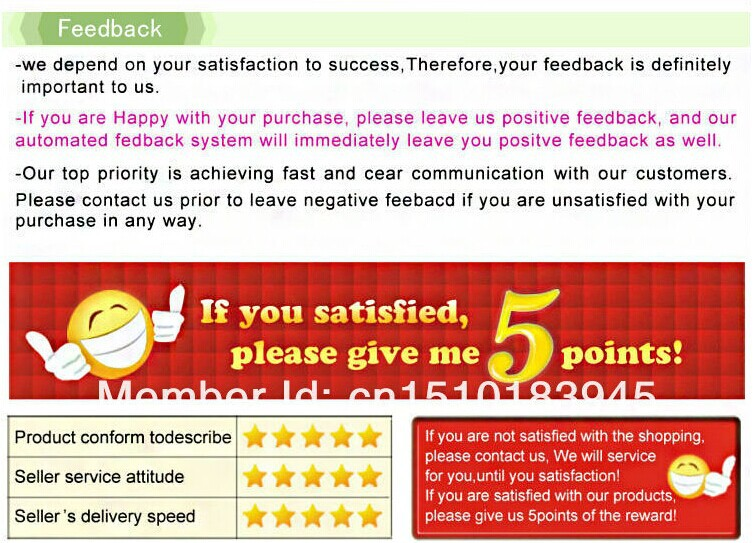## Write a review

Note: HTML is not translated!